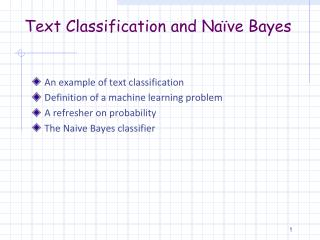DownloadDownload PresentationText Classification and Naïve Bayes

# Text Classification and Naïve Bayes

Download Presentation## Text Classification and Naïve Bayes

- - - - - - - - - - - - - - - - - - - - - - - - - - - E N D - - - - - - - - - - - - - - - - - - - - - - - - - - -
##### Presentation Transcript

1. Text Classification and Naïve Bayes • An example of text classification • Definition of a machine learning problem • A refresher on probability • The Naive Bayes classifier

3. Different ways for classification • Human labor (people assign categories to every incoming article) • Hand-crafted rules for automatic classification • If article contains: stock, Dow, share, Nasdaq, etc.  Business • If article contains: set, breakpoint, player, Federer, etc.  Tennis • Machine learning algorithms

4. What is Machine Learning? Definition: A computer program is said to learn from experience E when its performance P at a task T improves with experience E. Tom Mitchell, Machine Learning, 1997 • Examples: • Learning to recognize spoken words • Learning to drive a vehicle • Learning to play backgammon

5. Components of a ML System (1) • Experience (a set of examples that combines together input and output for a task) • Text categorization: document + category • Speech recognition: spoken text + written text • Experience is referred to as Training Data. When training data is available, we talk of Supervised Learning. • Performance metrics • Error or accuracy in the Test Data • Test Data are not present in the Training Data • When there are few training data, methods like ‘leave-one-out’ or ‘ten-fold cross validation’ are used to measure error.

6. Components of a ML System (2) Task • Type of knowledge to be learned (known as the target function, that will map between input and output) • Representation of the target function • Decision trees • Neural networks • Linear functions • The learning algorithm • C4.5 (learns decision trees) • Gradient descent (learns a neural network) • Linear programming (learns linear functions)

7. Defining Text Classification the document in the multi-dimensional space a set of classes (categories, or labels) the training set of labeled documents Target function: Learning algorithm: “Beijing joins the World Trade Organization”, China China

8. Naïve Bayes Learning Learning Algorithm: Naïve Bayes Target Function: The generative process: a priori probability, of choosing a category the cond. prob. of generating d, given the fixed c a posteriori probability that c generated d

9. A Refresher on Probability

10. Visualizing probability • A is a random variable that denotes an uncertain event • Example: A = “I’ll get an A+ in the final exam” • P(A) is “the fraction of possible worlds where A is true” Event space of all possible worlds. Its area is 1. Worlds in which A is true P(A) = Area of the blue circle. Worlds in which A is false Slide: Andrew W. Moore

11. Axioms and Theorems of Probability • Axioms: • 0 <= P(A) <= 1 • P(True) = 1 • P(False) = 0 • P(A or B) = P(A) + P(B) – P(A and B) • Theorems: • P(not A) = P(~A) = 1 – P(A) • P(A) = P(A ^ B) + P(A ^ ~B)

12. Conditional Probability • P(A|B) = the probability of A being true, given that we know that B is true H = “I have a headache” F = “Coming down with flu” P(H) = 1/10 P(F) = 1/40 P(H/F) = 1/2 F H Headaches are rare and flu even rarer, but if you got that flu, there is a 50-50 chance you’ll have a headache. Slide: Andrew W. Moore

13. Deriving the Bayes Rule Conditional Probability: Chain rule: Bayes Rule:

14. Back to the Naïve Bayes Classifier

15. Deriving the Naïve Bayes (Bayes Rule) and the document Given two classes We are looking for a that maximizes the a-posteriori (the denominator) is the same in both cases Thus:

16. Estimating parameters for the target function We are looking for the estimates and P(c) is the fraction of possible worlds where c is true. N – number of all documents Nc – number of documents in class c is a vector in the space where each dimension is a term: By using the chain rule: we have:

17. Naïve assumptions of independence • All attribute values are independent of each other given the class. (conditional independence assumption) • The conditional probabilities for a term are the same independent of position in the document. We assume the document is a “bag-of-words”. Finally, we get the target function of Slide 8:

18. Again about estimation For each term, t, we need to estimate P(t|c) Tct is the count of term t in all documents of class c Because an estimate will be 0 if a term does not appear with a class in the training data, we need smoothing: Laplace Smoothing |V| is the number of terms in the vocabulary

19. An Example of classification with Naïve Bayes

20. Example 13.1 (Part 1) Two classes: “China”, “not China” V = {Beijing, Chinese, Japan, Macao, Tokyo} N = 4

21. Example 13.1 (Part 1) Estimation Classification

22. Summary: Miscellanious • Naïve Bayes is linear in the time is takes to scan the data • When we have many terms, the product of probabilities with cause a floating point underflow, therefore: • For a large training set, the vocabulary is large. It is better to select only a subset of terms. For that is used “feature selection” (Section 13.5).